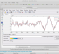## Sensor dataStarted by 2 years ago10 replieslatest reply 2 years ago183 views

I am involved in finding the directions of the received signals by an antenna array. For this I have a severe problem in which I am stuck. The 1st task was to find the DOAs of incoming signals for which I used the MUSIC algorithm and it was successful. Now I am to find the data x(t) if I know the covariance matrix Rxx of the sensor array. I tried it in Matlab as below but there is a problem. The problem is that when I check the Rzz matrix, its covariance is never identity matrix though literature says that it must be identity matrix. So where am I making a mistake?

clear all; close all; clc

Rxx= [3.1000 + 0.0000i  -0.7556 + 0.9467i   2.0871 - 1.6529i   0.8300 + 1.9440i   0.2419 - 1.7573i

-0.7556 - 0.9467i   3.1000 + 0.0000i  -0.7556 + 0.9467i   2.0871 - 1.6529i   0.8300 + 1.9440i

2.0871 + 1.6529i  -0.7556 - 0.9467i   3.1000 + 0.0000i  -0.7556 + 0.9467i   2.0871 - 1.6529i

0.8300 - 1.9440i   2.0871 + 1.6529i  -0.7556 - 0.9467i   3.1000 + 0.0000i  -0.7556 + 0.9467i

0.2419 + 1.7573i   0.8300 - 1.9440i   2.0871 + 1.6529i  -0.7556 - 0.9467i   3.1000 + 0.0000i];

[E,D]=eig(Rxx) % Eigen value decomposition

N=10; % number of snapshots. This is kept according to the size of Rxx

% Generating Gaussian complex random vector of zero mean and variance 1

z = 1/sqrt(2)*(rand(N, 1) +1i*rand(N,1));

xMean=mean(z); % For checking whether mean is zero or not

xVariance=var(z); % For checking whether variance is 1 or not

Rzz= z*z'/length(z); % This is just for verification that Rzz comes as an Identity matrix or not

% If Rzz comes out to be an identity matrix, then uncomment below line to

% find sampples of x(t)

%%%%%%%%%%%%%%%%%%%%%%%%%%%%%%%%%%

% xx=E*sqrt(D)*z;

[ - ]A sample covariance computed from 10 random samples is not going to be the identity matrix.

[ - ]Thank you very much for your response. You may be right. But I have a book written by a senior professor and he has said like this in his book. Let me share the 1st 11 pages of that book as a proof.

pages1-11.pdf

Now if you look at the last page of this pdf file and then look at eq.(12), it is the same where I am stuck. So, if you are right then why this senior professor has published this material?

[ - ]Your professor is absolutely correct. The key to eqn 12 is the expected value operator E. If you just generate a random z and compute zz^T, there is no guarantee that you will get an identity matrix. However, if you do that experiment many times and average the results, you will see that the answer will start getting closer to the identity matrix. The more you average, the closer you will get to identity (but remember, it will never be perfect).

It’s just the difference between the theory of statistics and it’s practical use (where you can only do a finite number of experiments, that’s all.

[ - ]Thank you very much for your explanation. You mean to say that the formulae of statistics are true only if we perform the experiment many times? Here is what I have tried but in vain:

clear all; close all; clc

N=10; % number of snapshots.

z = 1/2*(rand(N, 1) +1i*rand(N,1));

xMean=mean(z);

xVariance=var(z);

Rzz= z*z'/length(z);

Rzz=Round(Rzz)

When I run above program, I get Rzz as zero matrix not identity though I took 10 samples. Even if I increase it to 100 samples, still I get the same result.

[ - ]Hi,

Could you check this code;

clear all; close all; clc
N=100; % number of snapshots.
M = 3 % Number of dimensions/variables
Rzz = zeros(M, M)
for j=1:N
# z has N samples for 3 variables
z = (rand(N, M) +1i*rand(N,M));
xMean=mean(z);
xVariance=var(z);
z = z - mean(z);
Rzz += z'*z ;#/length(z);
end

Rzz=round(Rzz/N)

[ - ]Thank you very much for your reply. I ran your above code, but it gave me this error:

Error: File: FindingSamplesFromRxx.m Line: 6 Column: 3

The input character is not valid in MATLAB statements or expressions.

Then I replaced the symbol # by % and ran it, then it gave me this error:

Error: File: FindingSamplesFromRxx.m Line: 11 Column: 10

Character vector is not terminated properly.

Then I replaced the 2nd # symbol by % and ran it, again it gave me this error:

Error: File: FindingSamplesFromRxx.m Line: 11 Column: 10

Character vector is not terminated properly.

Now what to do to run it?

[ - ]I coded in Octave actually, I tested this in Matlab

clear all; close all; clc

N=100; % number of snapshots.

M = 3; % Number of dimensions/variables

Rzz = zeros(M, M);

for j=1:N

% z has N samples for 3 variables

z = (rand(N, M) +1i*rand(N,M));

xMean=mean(z);

xVariance=var(z);

z = z - mean(z);

Rzz = Rzz + z'*z ; %/length(z);

end

Rzz=round(Rzz/N)

[ - ]Thank you very much for your response. Yes, its ok now. But there is a logical problem wit this code. If you see my above code, I have tried to generate z as a column vector of size 100 x 1, but you have generated it as a matrix in your code i.e., 100 x 3. So if you also generate z as a column vector, then your code doesn't give Rzz as an identity matrix, but rather, it gives Rzz as a coulmn vector having same values.

[ - ]1 column would mean one random variable.  There won't be matrix for covariance for a single column data.

I commented in the code, you have missed them. 3 columns are for three variables, N is for the samples. Here covariance matrix would be then 3x3.

I hope you can understand it after studying the code.

[ - ]